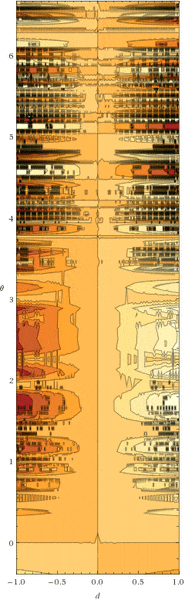# Help simplifying an equation

• B
Hi, just wondering if anyone could help me simplify (if possible) the following two equations.

x = sin((cos(((1-(cos(θ/2)^2))*360)/2)+1)/2*θ)*(d/2)

x = cos((cos(((1-(cos(θ/2)^2))*360)/2)+1)/2*θ)*d/2)*2

My math is pretty crap, so any help would be appreciated.

Thanks

## Answers and Replies

mfb
Mentor
Apart from some formatting and ##1-\cos^2(x) = \sin^2(x)## , there is not much to simplify. It has an interesting contour plot, however.

Apart from some formatting and ##1-\cos^2(x) = \sin^2(x)## , there is not much to simplify. It has an interesting contour plot, however.

Oh well, will have to use them in the current form. Thanks anyway.

If you don't mind me asking (as I really am crap at math) why is the contour plot interesting?

fresh_42
Mentor
Oh well, will have to use them in the current form. Thanks anyway.

If you don't mind me asking (as I really am crap at math) why is the contour plot interesting?
It looks like right from a seismograph or a piece of modern art.

member 587159
It looks like right from a seismograph or a piece of modern art.

Tbh, I didn't see anything special in it either.

fresh_42
Mentor
For the record (Source: https://www.wolframalpha.com/input/?i=sin((cos(((1-(cos(θ/2)^2))*360)/2)+1)/2*θ)*(d/2))•mfb
I typed your equation and "simplify" into wolframalpha, and this is what I got
It has a simpler looking form for x, d and theta real.

DrClaude
Mentor
It has an interesting contour plot, however.
My guess is that this is incorrect, as the 360 smells of degrees, leading to a much too rapid oscillation and a crappy contour plot. Change the 360 to 2*pi and you get something much more banal.

I typed your equation and "simplify" into wolframalpha, and this is what I got
It has a simpler looking form for x, d and theta real.
This is the same result as
##1-\cos^2(x) = \sin^2(x)##